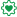# QlikView Creating Analytics

Discussion Board for collaboration related to Creating Analytics for QlikView.

Announcements
Coming your way, the Qlik Data Revolution Virtual Summit. October 27-29. REGISTER
cancel
Showing results for
Did you mean:
HighlightedNot applicable

## Problem with date and/or variable and/or WHERE condition

Good day,

I spent hours on a problem to finally discover that what I though my script will do wasn't right...

I have a table that contains all my data, having a date :

`TblData:LOAD * INLINE [MyID, MyDate1, 01/01/20102, 31/12/20103, 01/01/2011];`

I would like to create a table that contains the lines having the date lower than a specific day.

Here is the code I used :

`LET max_date = makedate(2011, 1, 1);TblResult_1:LOAD MyID AS ResID_1, MyDate AS ResDate_1RESIDENT TblDataWHERE num(MyDate) < num(\$(max_date));`

(I use the "num( )" function to avoid date format problems)

Unfortunatly, the script returns 0 lines (instead of 2 lines expected) !

After hours of search and tweeks, I found that this code :

`LET max_date = num(makedate(2011, 1, 1));TblResult_2:LOAD MyID AS ResID_2, MyDate AS ResDate_2RESIDENT TblDataWHERE num(MyDate) < \$(max_date);`

returns the 2 lines I expected...

The only difference is that the "num( )" function is in the variable declaration, instead of the request.

Why is there a difference between the results of my 2 scripts ?

1 Solution

Accepted Solutions
HighlightedEmployee

Hello,

LET evaluates the right part before storing (returning a date), then stores. \$(max_date) evaluates the contents of the variable, so if value assigned is "01/01/2011" the result of \$(max_date) should be 1 divided into 1 divided into 2011 which is equal to 0,000497, this is the value finally stored.

In the second case, LET evaluates Num(MakeDate()), which returns "40544". \$(max_date) evaluates 40544, which is equal to "40544" (the number itself)

So you are evaluating twice, when you only want once.

SET doesn't evaluate, just stores.

If you use SET instead of LET when you create the variable max_date, it will behave as you expect in the first case.

Hope that helps.

6 Replies
HighlightedEmployee

Hello,

LET evaluates the right part before storing (returning a date), then stores. \$(max_date) evaluates the contents of the variable, so if value assigned is "01/01/2011" the result of \$(max_date) should be 1 divided into 1 divided into 2011 which is equal to 0,000497, this is the value finally stored.

In the second case, LET evaluates Num(MakeDate()), which returns "40544". \$(max_date) evaluates 40544, which is equal to "40544" (the number itself)

So you are evaluating twice, when you only want once.

SET doesn't evaluate, just stores.

If you use SET instead of LET when you create the variable max_date, it will behave as you expect in the first case.

Hope that helps.

HighlightedNot applicable

This helps !

But why "LET = makedate(2011, 1, 1)" returns a string instead of a date ?

You say I "evaluate twice" because "\$( )" makes an evalution. But I'm forced to use this syntax in the request, right ?

HighlightedMVP

Hi,

TblData:

MyID, MyDate
1, 01/01/2010
2, 31/12/2010 // taking as String, because of MM/DD/YYYY format
3, 01/01/2011
];

AND

LET max_date = makedate(2011, 1, 1);

TblResult_1:
MyID AS ResID_1,
MyDate AS ResDate_1
RESIDENT TblData
WHERE num(MyDate) < num(\$(max_date)); // No need of Num () , simply replace it with

Where MyDate < '\$(max_date)'; // because it needs again a string comparison if not Number.

Regards, tresesco

HighlightedNot applicable

I always use "num( )" to surround my dates, to avoid date format problems (when I get dates from Excel or from DB, I often have a problem).

Thanx to you two, I found that I missed single quotes to make my variable a string.

`LET max_date = makedate(2011, 1, 1);...WHERE num(MyDate) < num('\$(max_date)');`

(but I still don't understand why it's not a date, but a string)

HighlightedEmployee

Hello,

`nmartin wrote:But why "LET = makedate(2011, 1, 1)" returns a string instead of a date ?`

Any value in QlikView is interpretable as literal or numeric. Since 01/01/2011 is interpretable as a numeric operation, is evaluable, and since LET evaluates before storing, this divides the result of the makedate function.

`nmartin wrote:You say I "evaluate twice" because "\$( )" makes an evalution. But I'm forced to use this syntax in the request, right ?`

That's why you need to do a SET instead of a LET, otherwise the reload will fail, because it will look for "max_date" as a field instead of a variable/value.

Hope that makes sense.

HighlightedEmployee

`nmartin wrote:(but I still don't understand why it's not a date, but a string)`

It was never a string since you used LET. For a string (literal) you should use SET.

Hope that helps.